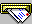Physikseite von B. Lampe# Research Interests and Activities

#### 4. QCD Effects

• Jets in e+e- Annihilation
• Heavy Quark Production in the Perturbative Regime

# 5. Substructure of Quarks and Leptons and a new Conception of the Universe

and the related problem of fermion families. Recently I have put forward a new idea, in which fermion and vector boson states are constructed from constituents ('tetrons'), which take the geometrical form of a tetrahedron or a cube. The model encodes all observed structures and phenomena of elementary particle physics in group theoretic items of the tetrahedral group which is isomorphic to the permutation group S4.

These ideas have finally been merged into a complete new conception of the physical world, whose main features can be described as follows:

I.

-Our universe is a 3-dimensional elastic substrate which once has condensed and now expands within some higher dimensional space (with dimension >=6).

-The elastic substrate is built from invisible 'tetron' constituents with bond length about the Planck length and binding energy the Planck energy.(*)

-All ordinary matter quarks and leptons are quasiparticle excitations gliding on the elastic tetron substrate.

-Since the quasiparticles fulfill Lorentz covariant wave equations, they perceive the universe as a 3+1 dimensional spacetime continuum lacking a preferred rest system.

-Any type of mass/energy induces curvature on the spacetime continuum as determined by the Einstein equations.

II.

-The 24 known quarks and leptons arise as excitations of a tetrahedral fiber structure, which is made up from 4 tetrons and extends into 3 additional 'internal' dimensions.(**)

-While the laws of gravity are due to the elastic properties of the substrate, particle physics interactions take place within the internal fibers, with the characteristic interaction energy being the Fermi scale.

-The masses of quarks and leptons can be determined from a Heisenberg type of Hamiltonian which describes the interactions of 2 neighboring tetrons.(***)

-3 of the quasiparticle excitations naturally have tiny masses, due to the conservation of total internal angular tetron momentum, and correspond to the 3 neutrino species.

-As a consequence of the free rotatability of the tetron isospins over each base point there is a local SU(2)xU(1) gauge symmetry.

-Electroweak spontaneous symmetry breaking takes place when neighboring tetron isospins align (freeze out) at energies/temperatures below the Fermi scale.

-Weak parity violation arises from the handedness of the internal tetrahedrons.

-Finally, Higgs fields and gauge bosons are excitations of bound tetron-antitetron pairs of neighboring fibers.

(*) Tetrons transform as the spinor representation 4 of SO(6) resp 8 of SO(6,1).
(**) Actually the tetrahedrons are formed by tetron isospin vectors and have a tetrahedral Shubnikov symmetry.
(***) The Hamiltonian can be written in terms of the tetron isospin vectors, see the enclosed review talk.

Details of the model like symmetry breaking, distribution of charges and mass generation are to be found in the following publications:

Space and Family, my first paper on the subject, contains the main idea and a geometrical interpretation, which is still relevant at this time e.g. for inclusion of gravity. Spacetime is considered discrete at very small distances, with only permutation symmetry transformations remaining relevant. When a fermion tetrahedron moves through a gravitational field, the directions of its 4 tetrons are given by the 4-bein which defines the gravitational field at that point.

World of Tetrons contains a first approach to the formation of vector bosons from tetrons

Chirality and Symmetry Breaking in a discrete internal Space, Internal space is interpreted as a vibrating 'crystal' or 'molecule' with pyritohedral symmetry, quarks and leptons being the normal modes of the vibrations.

Color and Isospin Waves from Tetrahedral Shubnikov Groups, an important supplement of the previous paper, in which internal spin waves are considered.

A microscopic Interpretation of the SM Higgs Mechanism, an extension to include the whole Standard Model symmetry breaking in the model

A Microscopic Approach to Quark and Lepton Masses and Mixings, an attempt to calculate the mass spectrum of quark and leptons. A newer and more elaborate calculation can be found HERE.Particle Physics and Cosmology in the Microscopic Model, a complete review which includes also an interpretation of gravity in the tetron model

Some Conclusions to be drawn from the Tetron Model concerning Cosmology and Fundamental Constants:
General Relativity from Elasticity of Tetron Bonds
Space and Time Dependence of Fundamental Constants
About the Value 1/137 of the Fine Structure Constant

... and here is the paper that summarizes the results: Dark Energy and the Time Dependence of Fundamental Particle Constants

My 2021 Talk at the 16th Marcel Grossmann Meeting in Rome gives a good overview on the subject.

Finally, I present my archives of how the tetron model has developed between 2007 and 2017, and in particular in the important years 2012, 2013 und 2014, and of how ich nahezu jeden Stein umgewendet habe, um den der Weisen zu finden:
2007 2008a 2008b 2009 2010 2011 2012/13 2013 2014a 2014b
2015/16
2018 QCD and the tetron model
2018 fundamental tetron interactions

### Biographical Notes## wissenschaftskritische Arbeiten

Index of my Homepage

Literature

Philosophy

A naive philosophers view on COSMOLOGYe-mail an:  Lampe.Bodo@web.de